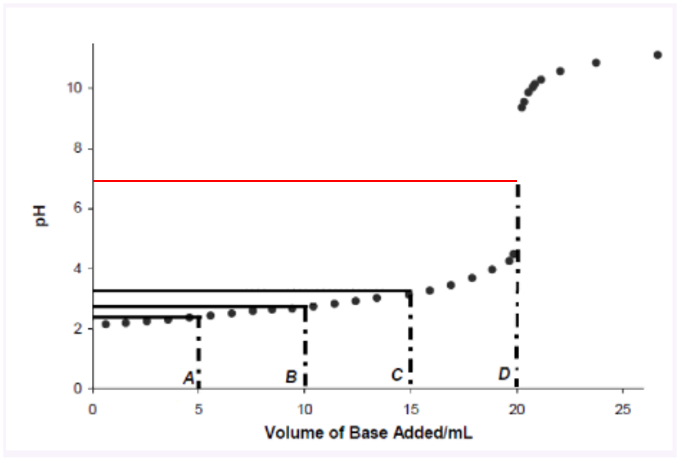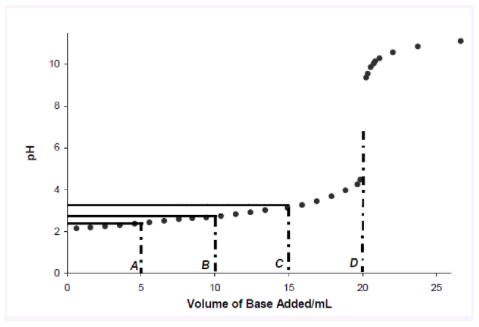# Problem: Using the equation pH = pKa + log{ [A-] / [HA] } and the graph, provide an estimate of the pKa of the acid using data from points A, B, and C. Report all 3 of the answers you calculate using this method as well as an average of the 3.

###### FREE Expert Solution

Based on the Henderson-Hasselbalch equation and the graph, we´re asked to provide an estimate of the pKa of the acid using data points A, B, and C, and an average of the 3.

The graph corresponds to a Titration curve, where a strong acid is neutralizing a strong base.

This is indicated by the equivalence point D, where the pH is around 7 (indicated by a red horizontal line).Remember that in the equivalence point, the volume of Acid and the volume of Base in solution are the same.

We determine pKa at each point with the following steps:

Step 1: Determine the approximate values of pH at the graph.

98% (22 ratings)###### Problem Details

Using the equation pH = pKa + log{ [A-] / [HA] } and the graph, provide an estimate of the pKa of the acid using data from points A, B, and C. Report all 3 of the answers you calculate using this method as well as an average of the 3.